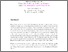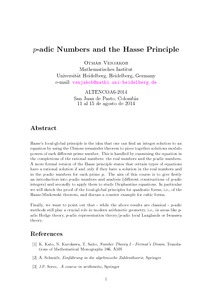# p-adic Numbers and the Hasse Principle

Benjakob, Otmar (2014) p-adic Numbers and the Hasse Principle. In: Altencoa6 - 2014, Agosto 11 al 15 de 2014, Universidad de Nariño - Colombia.Preview
Text
VENJAKOB.pdf

Download (304kB) | Preview

## Resumen

Hasse’s local-global principle is the idea that one can ﬁnd an integer solution to anequation by using the Chinese remainder theorem to piece together solutions modulo powers of each diﬀerent prime number. This is handled by examining the equation in the completions of the rational numbers: the real numbers and the p-adic numbers. A more formal version of the Hasse principle states that certain types of equations have a rational solution if and only if they have a solution in the real numbers and in the p-adic numbers for each prime p. The aim of this course is to give ﬁrstly an introduction into p-adic numbers and analysis (diﬀerent constructions of p-adic integers) and secondly to apply them to study Diophantine equations. In particular we will sketch the proof of the local-global principles for quadratic forms, i.e., of the Hasse-Minkowski theorem, and discuss a counter example for cubic forms. Finally, we want to point out that - while the above results are classical - p-adic methods still play a crucial role in modern arithmetic geometry, i.e., in areas like p- adic Hodge theory, p-adic representation theory/p-adic local Langlands or Iwasawa theory.

Tipo de Elemento: Conferencia o Taller artículo (Speech) p-adic numbers, hasse principle L Educación > LB Theory and practice of education > LB2300 Higher Education Facultad de Ciencias Exactas y Naturales > Programa de Licenciatura en Matemáticas > Eventos > Álgebra, Teoría de Números, Combinatoria y Aplicaciones Altencoa - 2014 Depto Matemáticas y Estadística 02 Sep 2014 14:10 02 Sep 2014 14:10 http://sired.udenar.edu.co/id/eprint/113Ver Elemento